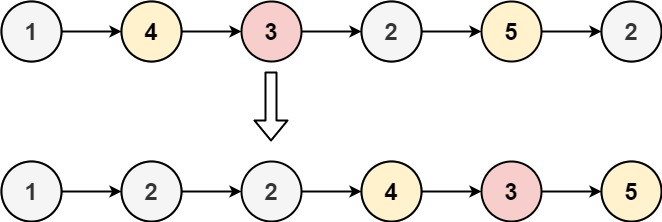## Algorithm

Problem Name: 86. Partition List

Given the `head` of a linked list and a value `x`, partition it such that all nodes less than `x` come before nodes greater than or equal to `x`.

You should preserve the original relative order of the nodes in each of the two partitions.

Example 1:```Input: head = [1,4,3,2,5,2], x = 3
Output: [1,2,2,4,3,5]
```

Example 2:

```Input: head = [2,1], x = 2
Output: [1,2]
```

Constraints:

• The number of nodes in the list is in the range `[0, 200]`.
• `-100 <= Node.val <= 100`
• `-200 <= x <= 200`

## Code Examples

### #1 Code Example with C Programming

```Code - C Programming```

``````
#include <stdio.h>
#include <stdlib>

struct ListNode {
int val;
struct ListNode *next;
};

struct ListNode* partition(struct ListNode* head, int x) {
if (head == NULL) return NULL;

struct ListNode *left, *right;
struct ListNode *lp, *rp;
struct ListNode *p;

left = (struct ListNode *)calloc(1, sizeof(struct ListNode));
right = (struct ListNode *)calloc(1, sizeof(struct ListNode));
lp = left;
rp = right;

while (p) {
if (p->val < x) {
lp->next = p;
lp = lp->next;
}
else {
rp->next = p;
rp = rp->next;
}
p = p->next;
}

lp->next = right->next;
rp->next = NULL;

free(left); free(right);

}

int main() {
struct ListNode *head = (struct ListNode *)calloc(6, sizeof(struct ListNode));
p->val = 1;
p->next = p + 1;
p = p->next;
p->val = 4;
p->next = p + 1;
p = p->next;
p->val = 3;
p->next = p + 1;
p = p->next;
p->val = 2;
p->next = p + 1;
p = p->next;
p->val = 5;
p->next = p + 1;
p = p->next;
p->val = 2;
p->next = NULL;

int x = 3;

while (p) {
printf("%d ", p->val);
p = p->next;
}
printf("\n");

return 0;
}
``````
Copy The Code &

Input

cmd
head = [1,4,3,2,5,2], x = 3

Output

cmd
[1,2,2,4,3,5]

### #2 Code Example with C++ Programming

```Code - C++ Programming```

``````
class Solution {
public:
ListNode* partition(ListNode* head, int x) {
ListNode left(0);
ListNode right(0);
ListNode* l = &left;
ListNode* r = &right;
while(cur){
if(cur->val < x){
l->next = cur;
l = l->next;
}
else{
r->next = cur;
r = r->next;
}
cur = cur->next;
}
r->next = NULL;
l->next = right.next;
return left.next;
}
};
``````
Copy The Code &

Input

cmd
head = [1,4,3,2,5,2], x = 3

Output

cmd
[1,2,2,4,3,5]

### #3 Code Example with Java Programming

```Code - Java Programming```

``````
class Solution {
public ListNode partition(ListNode head, int x) {
ListNode lessThan = null;
ListNode greaterThan = null;
if (lessThan == null) {
} else {
lessThan = lessThan.next;
}
lessThan.next = null;
} else {
if (greaterThan == null) {
} else {
greaterThan = greaterThan.next;
}
greaterThan.next = null;
}
}
}
}
}
``````
Copy The Code &

Input

cmd
head = [2,1], x = 2

Output

cmd
[1,2]

### #4 Code Example with Javascript Programming

```Code - Javascript Programming```

``````
const partition = function(head, x) {

// split list into two sides, left if val < x, else right
for (let node = head; node !== null; node = node.next) {
if (node.val < x) {
left.next = node;
left = node;
} else {
right.next = node;
right = node;
}
}

// combine the two sides
right.next = null;

};
``````
Copy The Code &

Input

cmd
head = [2,1], x = 2

Output

cmd
[1,2]

### #5 Code Example with Python Programming

```Code - Python Programming```

``````
class Solution:
else:
``````
Copy The Code &

Input

cmd
head = [1,4,3,2,5,2], x = 3

Output

cmd
[1,2,2,4,3,5]

### #6 Code Example with C# Programming

```Code - C# Programming```

``````
namespace LeetCode
{
public class _086_PartitionList
{
public ListNode Partition(ListNode head, int x)
{

while (p != null)
{
if (p.val < x)
{
lessP.next = p;
lessP = lessP.next;
}
else
{
greaterP.next = p;
greaterP = greaterP.next;
}
p = p.next;
}
greaterP.next = null;
}
}
}
``````
Copy The Code &

Input

cmd
head = [1,4,3,2,5,2], x = 3

Output

cmd
[1,2,2,4,3,5]